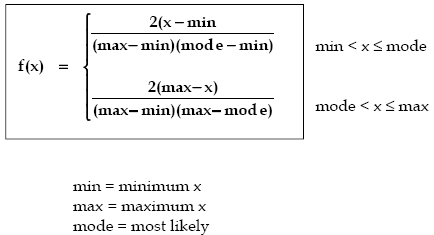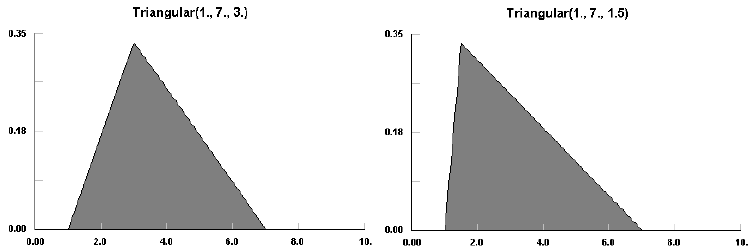AnyLogic

# triangularThe function automatically checks whether the most likely x value (mode) lies inside the specified (min, max) interval. In case it is greater than the specified maximum value, the function interprets it as the maximum - and vice versa: max is interpreted as mode, so the function call triangular (1, 5, 10) is the same as triangular (1, 10, 5): it generates a sample of the Triangular distribution with the min value : 1, max value: 10, and most likely value: 5.

The Triangular distribution is a continuous distribution bounded on both sides.

The Triangular distribution is often used when no or little data is available; it is rarely an accurate representation of a data set. However, it is employed as the functional form of regions for fuzzy logic due to its ease of use.

The Triangular distribution can take on very skewed forms including negative skewness. For the exceptional cases where the mode is either the min or max, the Triangular distribution becomes a right triangle.

### Samples### triangular(double min, double max, double mode)

Description
Generates a sample of the Triangular distribution. You can specify argument values in any order: (min, max, mode), or (min, mode, max). AnyLogic will automatically detect the argument with the maximum value, and set this value as the distribution's maximum. The same for most likely (mode) value. The function call triangular (1, 5, 10) is similar to triangular (1, 10, 5).
Parameters
Name Type Description
min double the minimum x value
max double the maximum x value
mode double the most likely x value
Result
Type Description
double the generated sample

### triangular(double min, double max)

Description
Generates a sample of the Triangular distribution with mode set to (min + max)/2. Is equivalent to triangular(min, (min + max)/2, max).
Parameters
Name Type Description
min double the minimum x value
max double the maximum x value
Result
Type Description
double the generated sample

### triangular(double min, double max, double mode, java.util.Random r)

Description
Generates a sample of the Triangular distribution using the specified random number generator. The function automatically checks whether the most likely x value (mode) lies inside the specified (min, max) interval. In the case it is greater than the specified maximum value, the function interprets it as the maximum - and vice versa: max is interpreted as mode, so the function call triangular (1, 5, 10, r) is the same as triangular (1, 10, 5, r): it generates a sample of the Triangular distribution with the min value:1, max value: 10, and most likely value: 5.
Parameters
Name Type Description
min double the minimum x value
max double the maximum x value
mode double the most likely x value
r java.util.Random the random number generator
Result
Type Description
double the generated sample

This document includes content from the Stat::Fit User's Manual. Copyright 2016 Geer Mountain Software Corp.